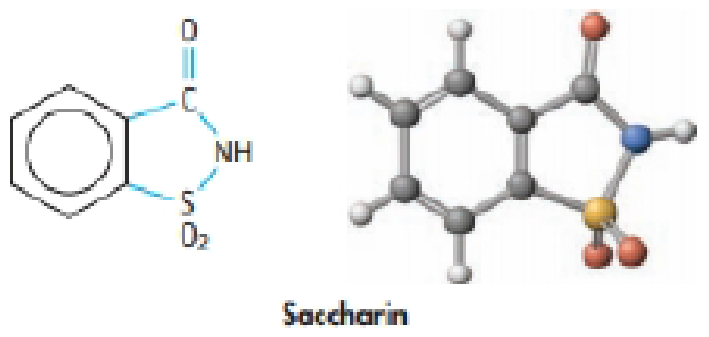# Saccharin (HC 7 H 4 NO 3 S) is a weak acid with p K a = 2.32 at 25 °C. It is used in the form of sodium saccharide, NaC 7 H 4 NO 3 S. What is the pH of a 0.01M solution of sodium saccharide at 25 °C?### Chemistry & Chemical Reactivity

9th Edition
John C. Kotz + 3 others
Publisher: Cengage Learning
ISBN: 9781133949640

#### Solutions

Chapter
Section### Chemistry & Chemical Reactivity

9th Edition
John C. Kotz + 3 others
Publisher: Cengage Learning
ISBN: 9781133949640
Chapter 16, Problem 97GQ
Textbook Problem
137 views

## Saccharin (HC7H4NO3S) is a weak acid with pKa = 2.32 at 25 °C. It is used in the form of sodium saccharide, NaC7H4NO3S. What is the pH of a 0.01M solution of sodium saccharide at 25 °C?Interpretation Introduction

Interpretation:

The pH of a 0.10M solution of sodium saccharide at 25oC has to be determined.

Concept introduction:

Equilibrium constants:

The equilibrium constant is used to quantitative measurement of the strength of the acid and bases in the water.

Ka is an acid constant for equilibrium reactions.

HA + H2OH3O++ A-Ka[H3O+][A-][HA]

Kb is base constant for equilibrium reaction.

BOH + H2OB++ OH-Ka[B+][OH-][BOH]

Ion Product constant for water

Kw= [H3O+][OH-]       =1.00×10-14 pH = -log[H3O+]pOH= -log[OH-]

Relation between pH and pOH

pH + pOH =14

### Explanation of Solution

The given pKa of saccharine HC7H4NO3S is 2.32.

Pka = -logKa2.32 = -logKaa= 10-2.32Ka = 4.8×10-3

Let’s calculate the Kb of saccharine.

Kw= Ka×Kb

Kb of C7H4NO3S- = KwC7H4NO3S-                              = 1.0×10-144.8×10-3                              = 2.1×10-12Kb of C7H4NO3S- is 2.1×10-12

The equilibrium chemical reaction is as follows.

C7H4NO3S-(aq) + H2O(l)HC7H4NO3S(aq) + OH-(aq)

The equilibrium expression:

Kb[HC7H4NO3S][OH-][C7H4NO3S-]

The initial concentration =0.10

Enter the ICE table the concentrations before equilibrium is established, the change that occurs as the reaction proceeds to equilibrium and the concentrations when equilibrium has been achieved

C7H4NO3S-(aq) + H2O(l)HC7H4NO3S(aq) + OH-(aq)I         0.10M                  --                    --                      --C           -x                      --                  + x                   + xE        (0

### Still sussing out bartleby?

Check out a sample textbook solution.

See a sample solution

#### The Solution to Your Study Problems

Bartleby provides explanations to thousands of textbook problems written by our experts, many with advanced degrees!

Get Started

Find more solutions based on key concepts
Which of the following foods is fare) rich in beta-carotene? sweet potatoes pumpkin spinach all of the above

Nutrition: Concepts and Controversies - Standalone book (MindTap Course List)

What is the structure and function of a mitochondrion?

Biology: The Dynamic Science (MindTap Course List)

How can solar flares affect Earth?

Horizons: Exploring the Universe (MindTap Course List)

List the differences between mitosis and meiosis in the following chart:

Human Heredity: Principles and Issues (MindTap Course List)

List three common substances that will sublime.

Chemistry for Today: General, Organic, and Biochemistry

Use geometry and Figure 38.17 (page 1227) to show these relationships: a. i = + and b. t = .

Physics for Scientists and Engineers: Foundations and Connections

A package is dropped at time t = 0 from a helicopter that is descending steadily at a speed i. (a) What is the ...

Physics for Scientists and Engineers, Technology Update (No access codes included)